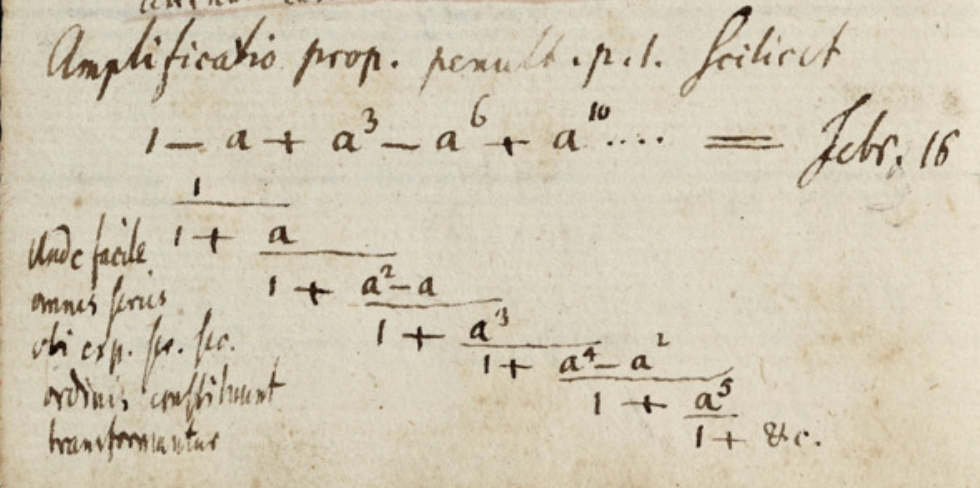# Divergent Series & Continued Fraction (from Gauss' Mathematical Diary)

Does someone have a reference or further explanation on Gauß' entry from May 24, 1796 in his mathematical diary (Mathematisches Tagebuch, full scan available via https://gdz.sub.uni-goettingen.de/id/DE-611-HS-3382323) on page 3 regarding the divergent series $$1-2+8-64...$$ in relation to the continued fraction $$\frac{1}1+\frac{\strut 2}1+\frac{\strut 2}1+\frac{\strut 8}1+\frac{\strut 12}1+\frac{\strut 32}1+\frac{\strut 56}1+128}}}}}}}$$

He states also - if I read it correctly - Transformatio seriei which could mean series transformation, but I don't see how he transforms from the series to the continued fraction resp. which transformation or rule he applied.

The OEIS has an entry (https://oeis.org/A014236) for the sequence $$2,2,8,12,32,56,128$$, but I don't see the connection either.

My question: Can anyone help or clarify the relationship that Gauss' used?

Torsten Schoeneberg remarked rightfully in the original question that the term in the series are $$(-1)^n\cdot 2^{\frac{1}{2}n(n+1)}$$ and Gerald Edgar conjectures it might be related to Gauss' Continued Fraction.

The entry from May 24, 1796 is worked out in a more general form on February 16, 1797 [reproduced below from this scan]$$1-a+a^3-a^6+a^{10}+\cdots=\frac{1}1+\frac{\strut a}1+\frac{\strut a^2-a}1+\frac{\strut a^3}1+\frac{\strut a^4-a^2}1+\frac{\strut a^5}{1+\cdots}}}}}}$$

so the coefficients alternate between $$a^{2n+1}$$ and $$a^{2n}-a^n$$.

Latin text: Amplificatio prop[ositionis] penult[imae] p[aginae] 1, scilicet $$\cdots$$
Unde facile omnes series ubi exp[onentes] ser[iem] sec[undi] ordinis constituunt transformantur.

Translation: Expanding on the proposition 1 from the next-to-last page $$\cdots$$
From here one can easily transform every series the exponents of which form a series of the second order.

These continued fractions of series of the form $$1+\sum_{n=1}^\infty a^{n(n+1)}-\sum_{n=1}^\infty a^{n^2}=\sum_{n=0}^\infty (-1)^n a^{n(n+1)/2}$$ are related to theta functions, see chapter 29 of "Series and Products from the Fifteenth to the Twenty-first Century". Apparently the series originated from Jakob Bernoulli (1690), see History of Continued Fractions and Padé Approximants.

• Great. Would you mind saying a bit more about the relation, and how to understand the continued fraction equality, for those who cannot see the google preview? Jun 26, 2019 at 21:19
• somehow the relevant page form that book is also missing from my Google preview... Jun 27, 2019 at 8:21